# Courses

Course: :
Teacher:

### BASIC   ▾  N : 25

Course nameNotePeriodTime
Table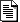Semester ISemester I

• Francesca MEROLA ( merola@mat.uniroma3.it - Dipartimento di Matematica e Fisica )

Semester I

• Marco CIANFRIGLIA ( marco.cianfriglia@uniroma3.it - Dipartimento di Matematica e Fisica )

Semester I

Semester ISemester II -

• Ugo BESSI ( bessi@mat.uniroma3.it - Dipartimento di Matematica e Fisica )

Semester II

• Marco PEDICINI ( marco.pedicini@uniroma3.it - Dipartimento di Matematica e Fisica )

Semester IIsemester IISemester II

• Francesco Cesarone ( francesco.cesarone@uniroma3.it - Universita degli Studi Roma TRE )Semester II

semester II

• Francesca MEROLA ( francesca.merola@uniroma3.it - Dipartimento di Matematica e Fisica )
• Filippo TOLLI ( filippo.tolli@uniroma3.it - Dipartimento di Matematica e Fisica )

semester II

• Vincenzo BONIFACI ( vincenzo.bonifaci@uniroma3.it - Dipartimento di Matematica e Fisica )

semester II

• Vito Michele ABRUSCI ( michele.abrusci@tlc.uniroma3.it, vitomichele.abrusci@uniroma3.it - Universita degli Studi Roma TRE )

Semester II

Semester II

• Roberto FERRETTI ( ferretti@mat.uniroma3.it - Dipartimento di Matematica e Fisica )

Semester II

• Severino Angelo Maria BUSSINO ( bussino@fis.uniroma3.it severinoangelomaria.bussino@uniroma3.it sbussino@uniroma3.it - Dipartimento di Matematica e Fisica )Semester II

### INTERMEDIATE   ▾  N : 12

Course nameNotePeriodTime
Table

Semester I

• Vincenzo BONIFACI ( vincenzo.bonifaci@uniroma3.it - Dipartimento di Matematica e Fisica )Semester II

• Luca SCHAFFLER ( luca.schaffler@uniroma3.it - Dipartimento di Matematica e Fisica )

Semester II

• Amos Turchet ( amos.turchet@uniroma3.it - Dipartimento di Matematica e Fisica )
HEIGHTS AND DIOPHANTINE EQUATIONS (TN520)

semester II

• Fabrizio BARROERO ( fabrizio.barroero@uniroma3.it - Dipartimento di Matematica e Fisica )

semester II

• Luciano TERESI ( luciano.teresi@uniroma3.it - Dipartimento di Matematica e Fisica )

semester II

• Roberto MAIELI ( maieli@uniroma3.it - Dipartimento di Matematica e Fisica )

Semester II

semester II

• Fabio LA FRANCA ( lafranca@fis.uniroma3.it - Dipartimento di Matematica e Fisica )
• Giorgio MATT ( matt@fis.uniroma3.it - Dipartimento di Matematica e Fisica )

### SPECIAL   ▾  N : 12

Course nameNotePeriodTime
Table
CLUSTER AND VIRAL EXPANSION: GENERAL DEFINITION AND CONVERGENCE CRITERIA (MINI-COURSE)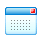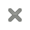• Roberto Fernandez ( - (NYU Shanghai) )
HANDS ON CONTINUUM MECHANICS MINI COURSE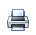#### HANDS ON CONTINUUM MECHANICS MINI COURSE

rganizers: the course is organized in partnership with three PhD Schools:
PhD on Mathematics, Dept. Mathematics & Physics, University Roma Tre;
PhD on Theoretical & Applied Mechanics, Dept. Aeronautical & Mechanical Engineering, Sapienza University, Roma;
PhD on Structural & Geotechnical Engineering, Dept. of Structural and Geotechnical Engineering, Sapienza
University, Roma.
Goal: understand the fundamentals of continuum mechanics through worked examples. Participants will tackle some
typical problems of continuum mechanics, and will learn to implement a given problem using the weak formulation into
the COMSOL software and to discuss the solution.
Synopsis of Lectures
1) Browse a model of nonlinear solid mechanics, from the implementation to the solution.
A first glance at the fundamentals of continuum mechanics: Kinematics, Constitutive, and Balance laws.
Differential form (strong) versus Integral form (weak).
2) Material Versus Spatial description.
A continuum body as a differentiable manifold.
Tell the difference between tensors: strain tensor versus stress tensor.
Pull back & push forward of scalar, vector and tensor fields.
Geometric elements; change of densities.
3) Solid mechanics versus Fluid mechanics
Kinematical constraints; isochoric motion.
Reference stress (Piola) & Actual stress (Cauchy).
Polar decomposition theorem; eigenspace of the stress tensor and of the strain tensor
4) Non linear solid mechanics
Worked example: large deformations of a hyperelastic solid under distortions. Target metric.
5) Material response
Worked example: from elastic energy to the constitutive law for the stress.
Transversely isotropic materials. Fiber reinforced materials.
Worked example: fiber reinforced hyperelastic solid under traction.
6) Fluid dynamics
Tackling Navier Stokes equations.
Worked examples: fluid in a channel; fluid around an obstacle.
7) Fluid-Structure interactions - theory
Worked examples: understand the moving mesh technique; how to write the problem of a beam immersed in a fluid.
8) Fluid-Structure interactions - practice
Worked example: implement and solve the problem of an oscillating beam immersed in a fluid• Luciano TERESI ( luciano.teresi@uniroma3.it - Dipartimento di Matematica e Fisica )
QUANTUM COMPLEXITY THEORY#### Quantum complexity theory

Course on quantum complexity theory and its relationship with classical complexity theory. We
introduce quantum computing theory, in a simplified way accessible to students who may have
never seen quantum mechanics before. Among the numerous advances in theoretical computer
science during the 20th century, three of them delineated the field: first, that there are
unsolvable computational problems (Turing's proof that the stopping problem is undecidable,
derived from Gödel’s incompleteness theorem). Second, solvable problems that can be solved
efficiently are likely a subclass of decidable ones. This is a consequence of the Cook-Levin
theorem, which generated the theory of NP-completeness and founded the field of complexity
theory. Third, some problems are not only difficult to solve exactly, but are also difficult to
solve approximately (the PCP theorem in the 1990s). Quantum complexity theory introduces
new complexity classes and many new questions to investigate. We consider several
separation results (proved or conjectured) between complexity classes (involving, for example,
NP, BPP, BQP).

• Marco PEDICINI ( marco.pedicini@uniroma3.it - Dipartimento di Matematica e Fisica )
TOPICS ON FANO VARIETIES 2: FOURFOLDS AND BEYOND.#### Topics on Fano varieties 2: fourfolds and beyond.

Abstract: We will survey some of the most recent progresses regarding the geography of Fano varieties of dimension 4 and higher, including, e.g, Kuechle's list, the 634 families of 4-folds constructed in flag varieties, and Fano varieties with special Hodge-theoretical properties (e.g. of K3 type)WHAT IS A FORCE?#### What is a Force?

The notion of force is ubiquitous in physics and the word "force" appears frequently in everyday life. Nevertheless, the notion of force is among the most subtle ones in physics.

The goal of this course is to go beyond the common working knowledge of forces that most of us have, and dwelve into the essence of this fundamental notion. Forces represent one of the major achievements of mathematical-physics, that is, the translation of the observations and the findings of experimental mechanics onto a mathematical model able to predict the outcome of mechanical phenomena.

Properly speaking, theoretical physics began with Newton's Principia, published in 1687;  at almost the same time mathematical analysis began. In this course, we shall review the key steps that brought to the development of the mathematical notions used to describe a force, and we shall describe the modern point of view of force modeling.

Contents
- Kinematics VS dynamics.
- Force as a probe for power
- The principle of virtual power
- Power VS Energy
- The principle of dissipation
- Invariance to change of observer

• Luciano TERESI ( luciano.teresi@uniroma3.it - Dipartimento di Matematica e Fisica )
GEOMETRY OF CANONICAL CURVES AND RELATED TOPICS#### GEOMETRY OF CANONICAL CURVES AND RELATED TOPICS

GEOMETRY OF CANONICAL CURVES AND RELATED TOPICS

Goals of the course
 Introduction to canonical models of algebraic curves of genus g and their
geometry.
 Construction of the canonical model for curves of low genus.
 Classical varieties through the canonical curve, re
ecting its geometry and
the properties of its Jacobian.
 Study of the family of canonical curves: discussion and examples in low
genus.
Program
 Linear series on curves: the canonical linear system.
 Canonical curves, linear series and rational normal scrolls.
 Quadrics through the canonical curve: theorems of Noether, Enriques,
Kempf.
 The Mukai constructions for canonical curves of low genus.
Prerequisites and references
 Basic notions on algebraic varieties.
 A main reference book for the topics in the course: Algebraic Curves I,
by E. Arbarello, M. Cornalba, Ph. Griths, J. Harris.
Further Information
 The course, of about 24 hours, will be given in the weeks of January and
February 2023, starting from January 9. Schedule of the lectures: Monday
and Wednesday 12 - 13.30, Room 311. Connection on line is possible.
 Possibly, variations of the schedule or special focusing on some of the themes
of the program will be de ned directly with the participants to the course.
sandro.verra@gmail.com , verra@mat.uniroma3.it

April - May 2023• Alessandro VERRA ( verra@mat.uniroma3.it - Dipartimento di Matematica e Fisica )
• Paola SUPINO ( supino@mat.uniroma3.it - Dipartimento di Matematica e Fisica )
DIOPHANTINE PROBLEMS#### Diophantine problems

The aim of this course is the study of certain diophantine problems with a stress on the use of height functions as a tool to prove finiteness of solutions of some Diophantine equation. We are going to start with an introduction on height functions and prove several useful properties of these objects. We are then going to apply these concepts on a couple of concrete classes of Diophantine equations. Finally, we are going to use heights to study the arithmetic of
polynomial dynamical systems, in particular their set of preperiodic points.

semester II

• Fabrizio BARROERO ( fabrizio.barroero@uniroma3.it - Dipartimento di Matematica e Fisica )
BRIDGELAND THEORY AND ITS APPLICATIONS#### Bridgeland Theory and its applications

t-structures and their hearts.
The space of Bridgeland stability conditions on a variety.
Stability conditions on K3 surfaces.
Construction of stability conditions.
The wall and chamber structure.
Gieseker stability and Bridgeland stability.
The Brill-Noether wall and the Gieseker wall.
Mukai’s program for K3-curves.
Ample divisors on Bridgeland moduli spaces.

March, April 2023COMPACTIFICATIONS OF MODULI SPACES#### Compactifications of moduli spaces

Abstract:
The space of parameters for a family of algebraic varieties of a fixed type is also an algebraic variety called moduli space. These are often at first not compact because the varieties being parametrized may degenerate in different ways acquiring singularities or becoming reducible. Therefore, it is essential for the theory to construct compactifications with good properties.
The main objective of this course is to give the basics of the stable pair compactification (also known as KSBA compactification), which generalizes the Deligne-Mumford-Knudsen compactification of the moduli space of n-pointed curves of genus g to moduli of higher dimensional algebraic varieties. In this generalization, the compactification parametrizes stable pairs: the datum of a variety X together with an effective Q-divisor D such that the pair (X,D) has semi-log canonical singularities and K_X+D is ample.
We will focus on the fundamental tools of this theory, concrete examples, and computations. Time permitting, we will also consider other compactification methods, especially the ones that come from geometric invariant theory and Hodge theory.

Nov, Dec 2022• Luca SCHAFFLER ( luca.schaffler@uniroma3.it - Universita degli Studi Roma TRE )
MATHEMATICAL QUANTUM MECHANICS#### Mathematical Quantum Mechanics

Abstract.
The first part of the course will present a rigorous analysis of solvable one-body problems in
non-relativistic quantum mechanics. More precisely, the spectral and dynamical features of the following
models will be examined: free motion in Rd, harmonic trap, hydrogen atom, zero-range potentials in
dimension d ? 3. The second part of the course will provide an introduction to mathematical scattering
theory. The time-dependent and time-independent approaches will be outlined, addressing the existence
and (asymptotic) completeness of wave operators. The definition of the scattering operator and its
connection to physical cross sections will be also discussed.

Prerequisites:
basic knowledge of Lebesgue theory and Lp spaces, Schwartz distributions and Sobolev
spaces, functional analysis and operators in Hilbert spaces.

Exam:
exposition and discussion of a research paper or textbook chapter.

borrowed from the course “FM450 - Aspetti Matematici della Meccanica
Quantistica” (Mathematical aspects of Quantum Mechanics) for the master degree in Mathematics.
Live streaming available (via Microsoft Teams). Whoever is interested in attending the course should
send an email to davide.fermi@polimi.it

Nov, Dec 2022• DAVIDE FERMI ( davide.fermi@polimi.it - Politecnico di Torino )
QUASI-PERIODIC DYNAMICS AND INVARIANT TORI: A GEOMETRIC VIEWPOINT#### Quasi-periodic dynamics and invariant tori: a geometric viewpoint

The dynamics of minimal translations on the torus give the simplest example of ergodic transformations. These so-called quasi-periodic dynamics (i.e. the super-position of finitely many oscillatory motions) are rich and show robustness properties in many dynamical systems of interest both in classical or statistical mechanics.

If the persistence, up to a small perturbation, of the fixed point of a diffeomorphism is generally obtained as a consequence of the classical implicit function theorem, this is not anymore the case for invariant manifolds of dimension greater than 0, which systematically persist only if they are “normally hyperbolic”, no matter what is the dynamics that they carry.  Of course, the question of persistence becomes far more delicate if one aims at controlling both the robustness of the manifold and the prescribed dynamics on it.
The study  of persistence and stability of invariant tori on which the dynamics is conjugated to a minimal translation, broadly goes under the name of “KAM theory” (after Kolmogorov, Arnold, and Moser). Starting from the 50’s, this theory unfolded in a wide spectrum of problems and conjectures, at the crossroads of geometry, symplectic topology and functional analysis.
In this course we shall give a more geometric and intrinsic viewpoint to quasi-periodic dynamics, their properties and the problem of their persistence.

Nov, Dec 2022THE ULTRAVIOLET PROBLEM FOR QED IN D=3 ( MINI-COURSE)#### The ultraviolet problem for QED in d=3 ( MINI-COURSE)

Abstract: We review some recent work on quantum electrodynamics on a three dimensional Euclidean spacetime, work which culminates in a proof of ultraviolet stability in a finite volume. The model is formulated on a fine lattice and bounds are obtained uniformly in the lattice spacing. The method is a renormalization group technique due to Balaban. Topics to be covered are (1.) Introduction, (2.) Block averaging for gauge fields, (3.) Block averaging for Fermi fields, (4.) Random walk expansions, (5.) Norms and polymer functions, (6.) Renormalization group with bounded gauge fields, (7.)
Renormalization, (8.) The full expansion.

Program: mon-wed-fri from oct 3 to oct 14, 2022, 14:00 --> 15:30; aula M1, Lungotevere Dante 376 (access also from L.go S. L. Murialdo 1), Dip.to Matematica e Fisica, Univ. Roma Tre

Oct, 2022• Prof. Jonathan Dimock, ( - Univ. Buffalo NY USA )

### IN OTHERS UNIVERSITIES   ▾

Questa pagina è disponibile in: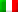Italiano